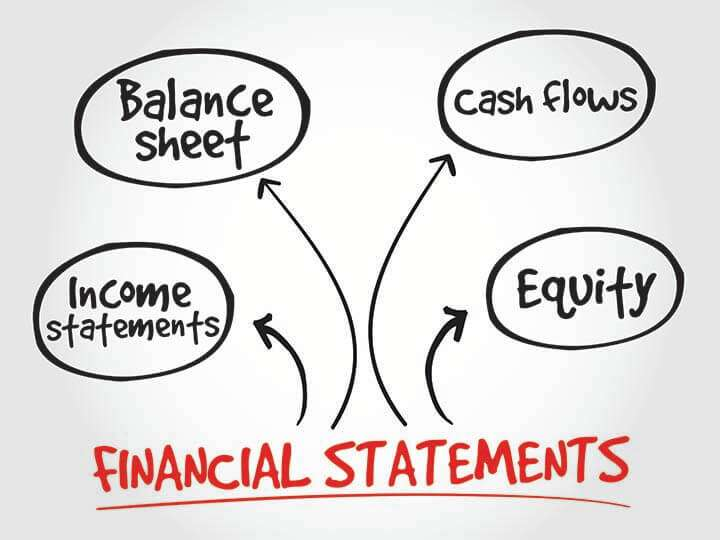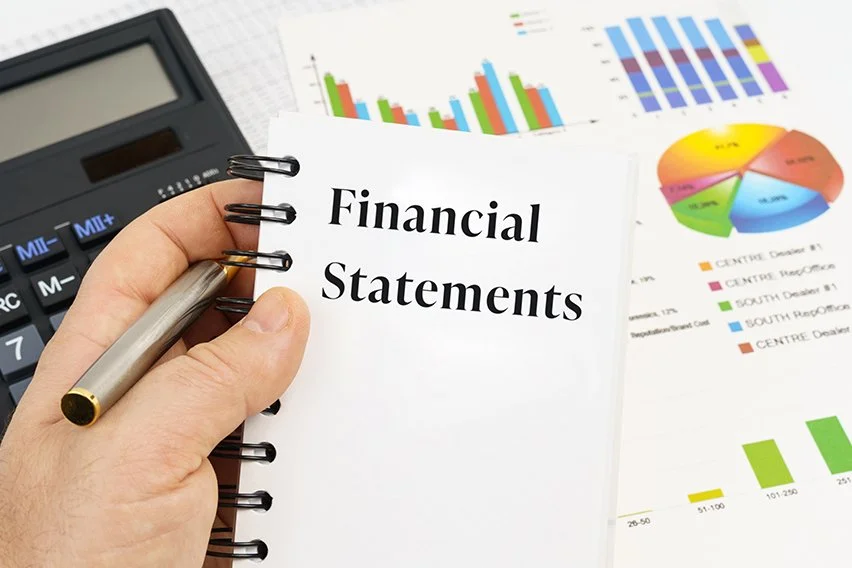8.8 C
New York
Friday, December 1, 2023

More

# Which Pair of Financial Statements Show Depreciation Expense?

Depreciation expense is a key component of a company’s financial statements, as it reflects the gradual wear and tear of a company’s fixed assets over time. There are two main financial statements that show depreciation expense: the balance sheet and the income statement.

The balance sheet is a snapshot of a company’s financial position at a specific point in time. It lists the company’s assets, liabilities, and equity. Depreciation expense is shown on the balance sheet as a reduction in the value of a company’s fixed assets. This is done through the process of depreciation, which is the allocation of the cost of a fixed asset over its useful life.The income statement, also known as the profit and loss statement, shows a company’s revenues, expenses, and net income over a specific period of time. Depreciation expense is shown on the income statement as a reduction in the value of a company’s fixed assets. This is done through the process of depreciation, which is the allocation of the cost of a fixed asset over its useful life.

## How Depreciation Expense is Calculated

There are several methods that can be used to calculate depreciation expense, including the straight-line method, the declining balance method, and the sum-of-the-years’-digits method. The choice of method depends on the nature of the asset and the company’s accounting policies.

The straight-line method is the most commonly used method for calculating depreciation expense. It involves dividing the cost of the asset by its useful life to determine the annual depreciation expense. For example, if a company buys a machine for \$100,000 and estimates its useful life to be 10 years, the annual depreciation expense would be \$10,000.The declining balance method involves calculating the depreciation expense for an asset at a higher rate in the earlier years of its useful life and a lower rate in the later years. The sum-of-the-years’-digits method involves calculating the depreciation expense for an asset based on the fraction of its useful life that has passed.

Conclusion:

In conclusion, depreciation expense is an important component of a company’s financial statements, as it reflects the gradual wear and tear of a company’s fixed assets over time. The two main financial statements that show depreciation expense are the balance sheet and the income statement. There are several methods that can be used to calculate depreciation expense, including the straight-line method, the declining balance method, and the sum-of-the-years’-digits method.

### Stay in touch

To be updated with all the latest news, offers and special announcements.